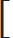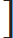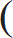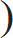# Profit and Loss - Aptitude test, questions, shortcuts, solved example videos

Video on Profit and Loss - shortcuts, tips and tricks

## Profit and Loss

Important Terms:

Cost Price (C.P.): Price at which at an article is purchased.
Selling Price (S.P.): Price at which at an article is sold
Profit/Gain: The seller is said to be in profit, if selling price (S.P.) is greater than cost price (C.P.)
Loss: The seller is said to be in loss, if selling price (S.P.) is lesser than cost price (C.P.)

Important Formulae:
 1) Loss% = Loss × 100 C.P.

 2) Profit% = Profit × 100 C.P.

3) When shopkeeper earns profit,
 Cost Price = 100 × S.P. - - - - - - (Given: Gain% and selling price of an article) (100 + Gain%)

 Selling Price = (100 + Gain%) × C.P. - - - - - - (Given: Gain% and cost price of an article) 100

4) When shopkeeper incurs loss,
 Cost Price = 100 × S.P. - - - - - - (Given: Loss% and selling price of an article) (100 - Loss%)

 Selling Price = (100 - Loss%) × C.P. - - - - - - (Given: Loss% and cost price of an article) 100

Quick Tips and Tricks

1) Profit = (S.P.) > (C.P.)

2) Loss = (S.P.) < (C.P.)

3) If profit earned by selling an article is 25 %, then S.P. = 125% of C.P.

4) If an article is sold at a loss of 30 %, then S.P. = 70 % of C.P.

5) A shopkeeper sells two similar items A and B. If A is sold at a gain of x % and B is sold at a loss of x %, then shopkeeper always incurs a loss given by:
 Loss% =Common loss and gain%2 =x2 10 10

6) A trader sells goods at cost price but uses a weight of x kg instead of y kg (false weights) and makes profit. This profit can be calculated using the formula shown below:

True weight – False weight = Error
 Gain% =Error × 100% (True weight – Error)

7) If cost price of X articles is equal to selling price of Y articles, then profit can be calculated using the formula:

a) C.P. of X = S.P. of Y
b) Number of X articles > Number of Y articles

 Profit% = No. of X articles – No. of Y articles × 100 No. of Y articles

8) If a seller makes X % above C.P. and offers a discount of Y%, then profit % or loss % can be calculated using the formula:
 Profit or Loss% = (X – Y) – X × Y 100

9) Discount:
 a) Discount% = Discount × 100 Marked Price (M.P.)
 b) If D1, D2, D3 are percentage of successive discounts on M.P., then S.P. = Marked Price1 – D11 – D21 – D3100 100 100
c) If a and b are two successive discount percentages, then single equivalent discount percentage is given as:
 Single equivalent discount percentage =(a + b) – ab100

Question Variety

Type 1: To find Profit/Loss or Selling Price/Cost Price.

Q 1. A shopkeeper sells an article for Rs. 200 with a loss of Rs. 20 %. Find the cost price of the article.

a. 220
b. 250
c. 280
d. 260
View solution

Correct Option: (b)

Hint:

 Cost Price = 100 × S.P. (100 – Loss%)
We are given that,
S.P. = Rs. 200 and loss = 20
 Cost Price = 100 × 200 (100 – 20)
 Cost Price = 100 × 200 80
Cost Price = Rs. 250

Q 2. A trader expects a gain of 15 % on his cost price. If in a week his sale is of Rs. 580, then what is his profit?

a. 75.65
b. 73.26
c. 72.50
d. 70.78
View solution

Correct Option: (a)

We are given selling price = Rs. 580 and expected profit of 15 %
Therefore, we can easily solve this numerical, considering basic formulae of profit and loss.
Let cost price = x
Selling price = C.P. + Profit
S.P. = C.P. + (15% of C.P.) ---------- (We know that profit is gained on cost price)
580 = x + (0.15 x)
580 = 1.15 x
Therefore,
x = 504.347
Cost Price = Rs. 504.35
Now, we have the cost price and hence,
Profit = S. P. – C.P. = 580 – 504.35 = Rs. 75.65
The trader gets a profit of Rs. 75.65

Alternate solution: This numerical can be easily solved using the trick specified below. Using such tricks reduce time consumed to solve the numerical.

Hint:

 Cost Price = 100 × S.P. (100 + Gain%)

 Cost Price = 100 × 580 (100 + 15)

 Cost Price = 100 × 580 = Rs. 504.35 (115)

Therefore,
Profit = C.P. – S.P.
C.P. = Rs.504. 35 and S.P. = Rs. 580
Profit = 580 – 504.35 = Rs. 75.65

The trader gets a profit of Rs. 75.65

Q 3. If a boy sells a book for Rs. 450 he gets a loss of 10 %, then find cost price. To gain 10 %, what should be the selling price?

a. 400, 500
b. 550, 600
c. 500, 550
d. 475, 525
View solution

Correct Option: (c)

1) Find cost price

Let C.P. of book = x and S.P. = Rs. 450
S.P. of book = C.P. – (10% of C.P.)
S.P. = x – (0.10x)
450 = 0.9 x
x i.e cost price = Rs. 500

2) Find Selling Price to gain 10 %.

Now, we are asked to find selling price to gain 10% profit.

Hint:

 Selling Price = (100 + Gain%) × C.P. 100

 Selling Price = (100 + 10) × 500 100

 Selling Price = (110) × 500 100

Therefore, selling Price = Rs. 550

Q 4. A merchant sells 30 metres of cloth and gains selling price of 10 metres. Find the gain percent.

a. 15 %
b. 25 %
c. 50 %
d. 75%
View solution

Correct Option: (c)

Here, selling price of 10 m cloth is obtained as profit.
Profit of 10 m cloth = (S.P. of 30 m cloth) – (C.P. of 30 m cloth)
Selling price of 20 m cloth = Selling Price of 30 m of cloth
Let cost of each metre be Rs. 100.
Therefore, cost price of 20 m cloth = Rs. 2000 and S.P. of 20 m cloth = Rs. Rs. 3000

 Profit% = 10 × 100 = 50% 20

Profit of 50 % was made by the merchant.

Type 2: Calculate profit/loss If cost price of X articles is equal to selling price of Y articles. (C.P. of X articles = S.P. of Y articles)

Q 5. S.P. of 10 candles is same as C.P. of 12 candles. Find the gain percent.

a. 11 %
b. 15 %
c. 20 %
d. 25 %
View solution

Correct Option : (c)

(Number of Y articles > Number of X articles)

Hint:

 Profit% = No. of X articles – No. of Y articles × 100 No. of Y articles
No. of X articles = 10
No. of Y articles = 12
Therefore,
 Profit% = 12 – 10 × 100 = 200 10 10
Profit % = 20 %

Q 6. The selling price of 40 apples is equal to cost price of 35 apples. Find the profit or loss obtained.

a. Gain of 5.5 %
b. Gain of 12.5 %
c. Loss of 5.5 %
d. Loss of 12.5 %
View solution

Correct Option: (d)

Let C.P. of each apple be Re 1/-.
Therefore,
C.P. of 40 apples = Rs. 40
S.P. of 40 apples = Rs. 35
C.P. of 40 apples > S.P. of 40 apples
Loss = 40 – 35 = Rs. 5

 Loss% = Loss × 100 C.P.
 Loss% = 5 × 100 = 12.5% 40

Type 3: Find loss incurred by the shopkeeper
a) Material A sold at x % gain and material B sold at x % loss.
b) Material A sold at x % gain and material B sold at y % loss.

Q 7. A man purchased two plots for Rs. 5,00,000. On one he gains 15 % while on the other he losses 15%. Find how much does he gain or lose in the transaction.

a. 1.5 %
b. 2 %
c. 2.25 %
d. 2.50 %
View solution

Correct Option: (c)

Generally in such cases, there is always loss.
So always remember, when two materials are sold and if one material gets profit and the other gets a loss, then use the trick shown below to calculate the loss.

 Loss% =Common loss and gain%2 =x2 10 10

Therefore, here common loss and gain % = 15%
Hence,
 Loss% =152 = 2.25% 10

Q 8. A boy bought camel and carriage for Rs. 5000. He sells the camel at a gain of 20% and the carriage at a loss of 10%. If he gains 3% on the whole, then find the cost of the camel.

a. 2170
b. 2400
c. 2315
d. 2600
View solution

Correct Option: (a)

Now, in this numerical, there is no common loss and gain %.
Hence, solve it making equations.

Let cost price of camel be x.
As cost of camel and carriage = Rs 5000
Cost of carriage = Rs. (5000 – x)
After selling camel he gains 20% and on carriage a loss of 10%. But on the whole he gains 3%.

Therefore,
20% of x – 10 % of (5000 – x) = 3 % of 5000

 20 × x – 10 × (5000 – x) = 3 × 5000 100 100 100
 x – (5000 – x) = 150 5 10
 10x – (5000 – x) × 10 = 150 × 10 5 10
2x-5000+x=1500
3x=1500+5000
x=2166.67

The cost of camel = Rs. 2166.67
Option (a) is the correct answer

Q 9. A man sells one camera A for Rs. 7500 at a gain of 20% and another camera B for Rs. 8550 at a loss of 5 %. Find his total loss or gain%.

a. 2.7 %
b. 5.2 %
c. 4.2 %
d. 5.1 %
View solution

Correct Option: (b)

Here, we just know the selling price and the gain and loss incurred, on two cameras.
Therefore, first calculate the cost price of both the cameras, because gain or loss is calculated on the cost price of the material.
Hint:

 When shopkeeper earns profit, Cost Price = 100 × S.P. (100 + Gain%)
 When shopkeeper incurs loss, Cost Price = 100 × S.P. (100 – Loss%)
 C.P. of camera A = 100 × 7500 = 100 × 7500 = Rs. 6250 (100 +20) 120
 C.P. of camera B = 100 × 8550 = 100 × 8550 = Rs. 9000 (100 – 5) 95

Total C.P. = Cost of camera A + Cost of camera B
Total C.P. = 6250 + 9000 = Rs. 15250
Total S.P. = 7500 + 8550 = Rs. 16050

Selling Price > Cost Price, hence man gains during this transaction.
Gain = S.P. – C.P. = 16050 – 15250 = Rs. 800
 Gain% = Gain × 100 C.P.
 Gain% = 800 × 100 = 5.24% 15250

Type 4: Trader sells goods at cost price but uses a weight of x kg instead of y kg (false weights) and makes profit. Calculate profit.

Q 10. A shopkeeper sells his goods at cost price but uses a weight of 970 grams for a kg. weight. What is his gain percent?

a. 5.08 %
b. 4.23 %
c. 3.26 %
d. 3.09 %
View solution

Correct Option: (d)

 Gain% =Error × 100% (True weight – Error)

Error = True weight – False weight
Error = 1000 – 970 = 30
 Gain% =30 × 100% (1000 – 30)
Gain %= 3.09%

Q 11. A dishonest shopkeeper sells his grocery using weights 10 % less than true weights and makes a profit of 30 %. Find his total gain percentage.

a. 49.4 %
b. 44.44 %
c. 55.55 %
d. 39.88 %
View solution

Correct Option: (b)

Let weight of grocery bag be 1000 gm.
Now, the shopkeeper sells his grocery using weights 10 % less than true weights.
Hence, actual weight of bag = 90 % of 1000 gm = 900 gm
If each gram = Re.1, C.P. of each bag containing 900 gm = Rs. 900
The shopkeeper sells with a gain of 30 % on true C.P.

Calculate the S.P.

 Selling Price = (100 + Gain%) × C.P. 100
Therefore,
 Selling Price = 130 × Rs. 1000 = Rs. 13000 100

Gain = S.P. – C.P. = 1300 – 900 = Rs. 400
 Gain% = 400 × 100 = 44.44% 900

 Q 12. A dealer sells his goods at cost price. If by using false weights he gains 4 8 %, then find the weight he uses for 1 kg. 23

a. 968.53 gm
b. 992.56 gm
c. 958.34 gm
d. 950.50 gm
View solution

Correct Option: (c)

 Gain% =Error × 100% (True weight – Error)

 We are given that, dealer gains 4 8 % after selling the goods at cost price. 23

Let error be x.
 4 8 % =x × 100% 23 (1000 – x)
 100 =100x% 23 (1000 – x)
(1000 – x) = 23 x
1000 = 24 x
x = 41.66

False weight = True weight – Error
False weight = 1000 – 41.66 = 958.34 gms

The false weight used by the dealer is 958.34 grams.

Type 5: Numerical on Discount and Successive discounts/profit

Q 13. After two successive discounts, a tie with a list price of Rs. 120 is available at Rs. 90. If second discount is 9 %, what is the first discount?

a. 15.23 %
b. 13.26 %
c. 17.58 %
d. 18.53 %
View solution

Correct Option: (c)

Let first discount = x
91 % discount of (100 – x) % of 120 = 90

 91 × (100 – x) × 120 = 90 100 100
 (100 – x) = (90 × 100 × 100) = 82.42 (120 × 91)
x = (100 – 82.42) = 17.58

Therefore, first discount = 17.58 %

Q 14. Find the single discount equivalent to a series discount of 30 %, 20 % and 10 %.

a. 48.3 %
b. 49.6 %
c. 38.21 %
d. 33.33 %
View solution

Correct Option: (b)

Let marked price be Rs. 100
Therefore, selling price = 90%, 80% and 70% of Rs. 100

 Selling Price = 90 × 80 × 70 × 100 = 50.4 100 100 100
Selling Price = Rs. 50.4

Required discount = Marked Price – Selling Price
= 100 – 50.4
= 49.6 %

Q 15. A dealer marks price of all the goods at 30 % above the cost price and assumes that he will make a profit of 15 % if he offers a discount of 15%. Find what will be his actual profit on sales?

a. 15 %
b. 30 %
c. 12.50 %
d. 10.50 %
View solution

Correct Option: (d)

Let cost price goods be Rs. 100
Marked price (Selling Price) marked by the shopkeeper on goods = Rs. 130
He sells the goods at a discount of 15 %
Therefore,
Selling price = 85 % of Rs. 130 = Rs. 110.50
Gain = S.P. – C.P. = 110.5 – 100 = 10.50 %

Alternate solution:
He sells the goods at a discount of 15 %
15% discount on Rs. 130 = Rs. 19.50
Selling Price = Marked Price – Discount = 130 – 19.50 = Rs. 110.50

Q 16. A manufacturer sells a pair shoes to a wholesale dealer at a profit of 20 %. Wholesaler sells them to retailer at a profit of 25 %. The shoes are again sold to the customer for Rs. 50.50, therby earning a profit of 30 %. Find the cost price of manufacturer.

a. Rs. 20.36
b. Rs. 22.90
c. Rs. 25.89
d. Rs. 30.50
View solution

Correct Option: (c)

Profit earned by manufacturer = 20 %
Profit earned by wholesaler = 25 %
Profit earned by retailer = 30%
S.P. of shoes = Rs. 50
Therefore, 130 % of 125 % of 120 % of x = 50.50

 = 120 × 125 × 130 × x = 5050 100 100 100 100
 195 x = 5050 100 100
 x = 5050 × 100 195 × 100
x = 25.89
Cost price of shoes = Rs. 25.89

Average - Aptitude test, questions, shortcuts, solved example videos
Average - Quantitative aptitude tutorial with easy tricks, tips, short cuts explaining the concepts. Online aptitude preparation material with practice question bank, examples, solutions and explanations. Video lectures to prepare quantitative aptitude for placement tests and competitive exams like MBA, Bank exams, RBI, IBPS, SSC, SBI, RRB, Railway, LIC, MAT. Very useful for freshers, engineers, software developers taking entrance exams. Learn and take practice tests!
Problem on Ages - Aptitude test, questions, shortcuts, solved example videos
Problems on Ages - Quantitative aptitude tutorial with easy tricks, tips, short cuts explaining the concepts. Online aptitude preparation material with practice question bank, examples, solutions and explanations. Video lectures to prepare quantitative aptitude for placement tests and competitive exams like MBA, Bank exams, RBI, IBPS, SSC, SBI, RRB, Railway, LIC, MAT. Very useful for freshers, engineers, software developers taking entrance exams. Learn and take practice tests!
Compound Interest - Aptitude test, questions, shortcuts, solved example videos
Compound Interest - Here we have sample solved questions, tests, tips, tricks and formulae on Compound Interest. This is useful for campus interview, competitive examination and various entrance tests.